## Introduction

A list of abbreviations and symbols is common in many scientific documents. These types of lists can be created with LaTeX by means on the nomencl package. This article explains how to create nomenclatures, customizing the ordering and subgrouping of the symbols.

Nomenclature entries work pretty much like index entries:

\documentclass{article}
\usepackage{nomencl}
\makenomenclature

\begin{document}
Here is an example:
\nomenclature{$$c$$}{Speed of light in a vacuum}
\nomenclature{$$h$$}{Planck constant}

\printnomenclature
\end{document}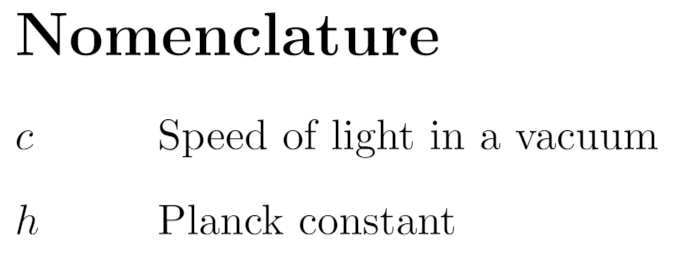As usual the package is imported in the preamble by \usepackage{nomencl}. The three basic commands to produce the nomenclatures are:

• \makenomenclature. Usually put right after importing the package.
• \nomenclature. Used to define the nomenclature entries themselves. Takes two arguments, the symbol and the corresponding description.
• \printnomenclatures. This command will print the nomenclatures list.

## Basic Syntax

Additional options can be used when importing the nomencl package. The next example shows how to add the nomenclature to the table of contents and how to change the default language:

\documentclass{article}
\usepackage[spanish]{babel}
\usepackage[intoc, spanish]{nomencl}
\makenomenclature

\begin{document}
\tableofcontents

\section{Primera Sección}

Lorem ipsum dolor sit amet, consectetuer adipiscing elit. Etiam lobortisfacilisis sem. Nullam nec mi et neque pharetra sollicitudin. Praesent imperdie...

\nomenclature{$$c$$}{Speed of light in a vacuum}
\nomenclature{$$h$$}{Planck constant}

\printnomenclature
\end{document}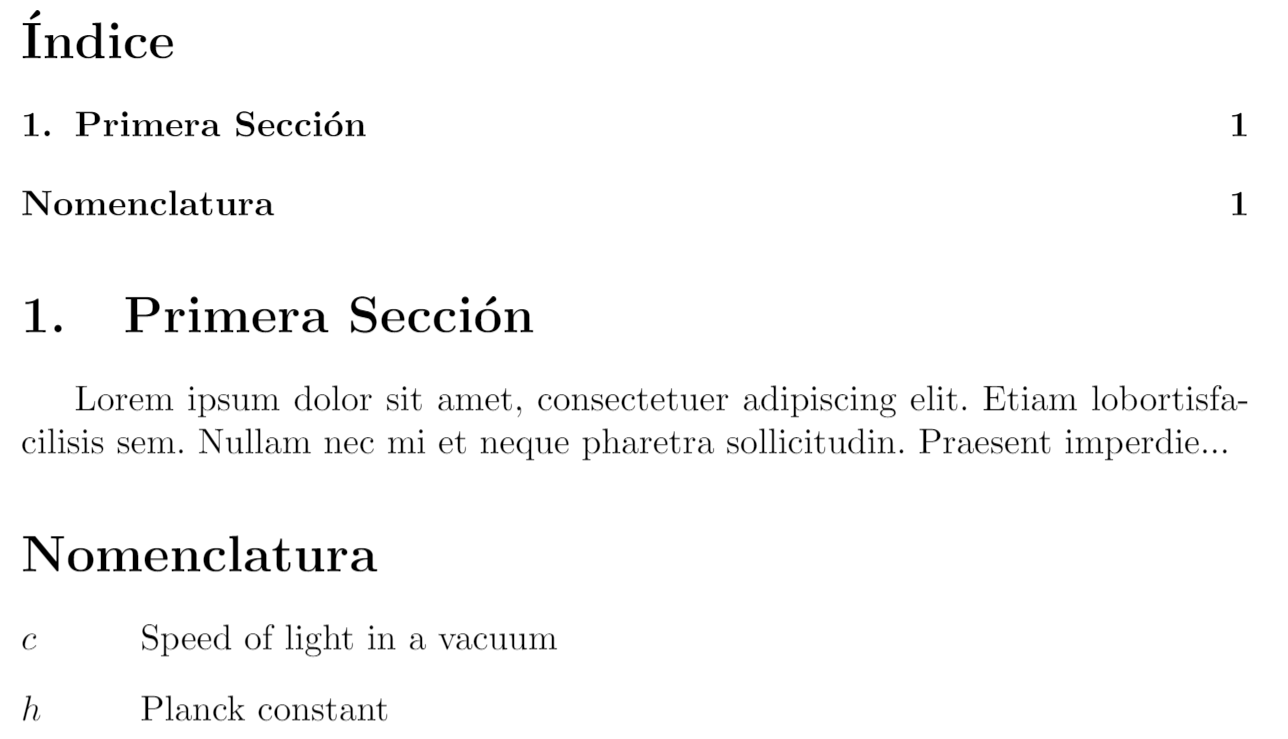The additional options used here in when importing the package are:

• intoc Adds the Nomenclature to the table of contents.
• spanish Changes the language, translating the default title Nomenclatures accordingly. The supported languages are: croatian, danish, english, french, german, italian, polish, portuguese, russian, spanish and ukranian.

Other useful features of the nomencl package are the possibility of manually setting the nomenclature title, and adding an additional annotation.

\documentclass{article}
\usepackage{nomencl}
\makenomenclature

\renewcommand{\nomname}{List of Symbols}

\renewcommand{\nompreamble}{The next list describes several symbols that will be later used within the body of the document}

\begin{document}
Here is an example:

\nomenclature{$$c$$}{Speed of light in a vacuum}
\nomenclature{$$h$$}{Planck constant}

\printnomenclature
\end{document}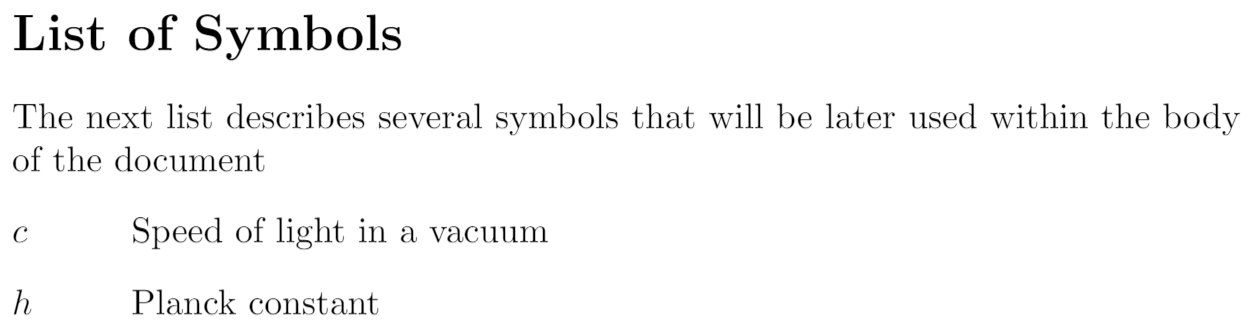The line

\renewcommand{\nomname}{List of Symbols}

changes the default title.

The command

\renewcommand{\nompreamble}{The next list...}

inserts some text in between the title and the list symbols.

## Grouping

To group the symbols depending on their type some additional work is needed. We add a prefix to each symbol and use the etoolbox package to compare the prefixes.

\documentclass{article}
\usepackage{amssymb}
\usepackage{nomencl}
\makenomenclature

%% This code creates the groups
% -----------------------------------------
\usepackage{etoolbox}
\renewcommand\nomgroup{%
\item[\bfseries
\ifstrequal{#1}{P}{Physics constants}{%
\ifstrequal{#1}{N}{Number sets}{%
\ifstrequal{#1}{O}{Other symbols}{}}}%
]}
% -----------------------------------------

\begin{document}
Here is an example:

\nomenclature[P]{$$c$$}{Speed of light in a vacuum}
\nomenclature[P]{$$h$$}{Planck constant}
\nomenclature[P]{$$G$$}{Gravitational constant}
\nomenclature[N]{$$\mathbb{R}$$}{Real numbers}
\nomenclature[N]{$$\mathbb{C}$$}{Complex numbers}
\nomenclature[N]{$$\mathbb{H}$$}{Quaternions}
\nomenclature[O]{$$V$$}{Constant volume}
\nomenclature[O]{$$\rho$$}{Friction index}

\printnomenclature
\end{document}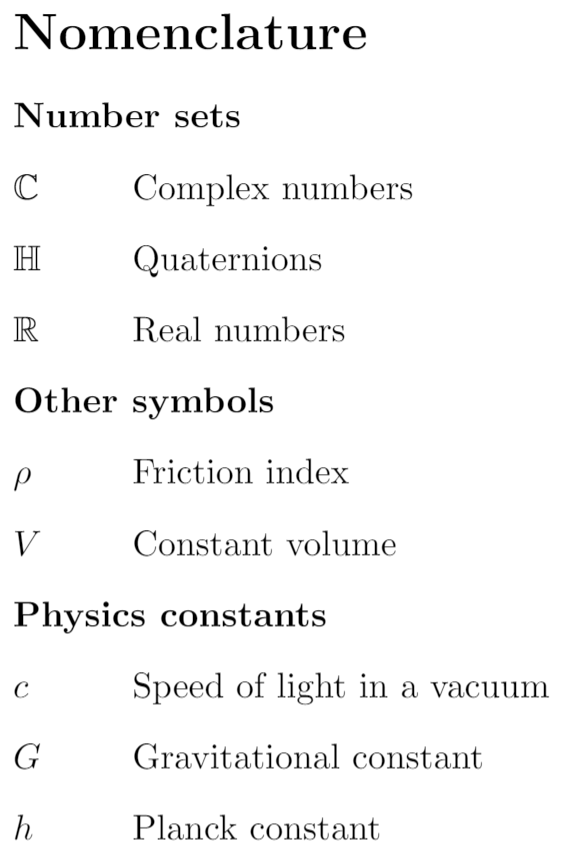Some extra groups are added. The code for this is not that simple, it uses the command \ifstrequal{}{}{}{}, the first two arguments are the strings to compare, if they are equal the term is added to the group, otherwise the next nested condition is checked.

Notice that now each \nomenclature command has an additional argument, the prefix, inside brackets; which is used in the grouping code.

If etoolbox is not available one can use the ifthen package instead, which provides the conditional \ifthenelse{}{}{}, but the syntax is slightly more complex:

\usepackage{ifthen}
\renewcommand{\nomgroup}{%
\item[\bfseries
\ifthenelse{\equal{#1}{P}}{Physics constants}{%
\ifthenelse{\equal{#1}{O}}{Other symbols}{%
\ifthenelse{\equal{#1}{N}}{Number sets}{}}}%
]}


This will produce the same nomenclature groups.

## Adding units for physical constants

Another interesting feature is using the siunitx package to add units, aligning them to the right of the corresponding entries. For this, one has to define the nomunit macro as shown in the following example:

\documentclass{article}
\usepackage{amssymb}
\usepackage{nomencl}
\usepackage{siunitx}
\usepackage{hyperref}
\makenomenclature
\hypersetup{
urlcolor=blue,
}
%% This will add the subgroups
%----------------------------------------------
\usepackage{etoolbox}
\renewcommand\nomgroup{%
\item[\bfseries
\ifstrequal{#1}{A}{Physics Constants}{%
\ifstrequal{#1}{B}{Number Sets}{%
\ifstrequal{#1}{C}{Other Symbols}{}}}%
]}
%----------------------------------------------

%% This will add the units
%----------------------------------------------
\newcommand{\nomunit}{%
\renewcommand{\nomentryend}{\hspace*{\fill}#1}}
%----------------------------------------------

\title{Nomenclatures Example}
\author{Overleaf Team}
\date{\today}

\begin{document}
\maketitle

\noindent This is an example to show how the \texttt{nomencl} package works, with units typeset via the \texttt{siunitx} package. \href{https://www.nist.gov/pml/fundamental-physical-constants}{NIST} was referenced to provide the values of physical constants, and their corresponding units---links are provided via the \texttt{hyperref} package:

\nomenclature[A, 02]{$$c$$}{\href{https://physics.nist.gov/cgi-bin/cuu/Value?c}
{Speed of light in a vacuum}
\nomunit{\SI{299792458}{\meter\per\second}}}
\nomenclature[A, 03]{$$h$$}{\href{https://physics.nist.gov/cgi-bin/cuu/Value?h}
{Planck constant}
\nomunit{\SI[group-digits=false]{6.62607015e-34}{\joule\per\hertz}}}
\nomenclature[A, 01]{$$G$$}{\href{https://physics.nist.gov/cgi-bin/cuu/Value?bg}
{Gravitational constant}
\nomunit{\SI[group-digits=false]{6.67430e-11}{\meter\cubed\per\kilogram\per\second\squared}}}
\nomenclature[B, 03]{$$\mathbb{R}$$}{Real numbers}
\nomenclature[B, 02]{$$\mathbb{C}$$}{Complex numbers}
\nomenclature[B, 01]{$$\mathbb{H}$$}{Quaternions}
\nomenclature[C]{$$V$$}{Constant volume}
\nomenclature[C]{$$\rho$$}{Friction index}

\printnomenclature

\end{document}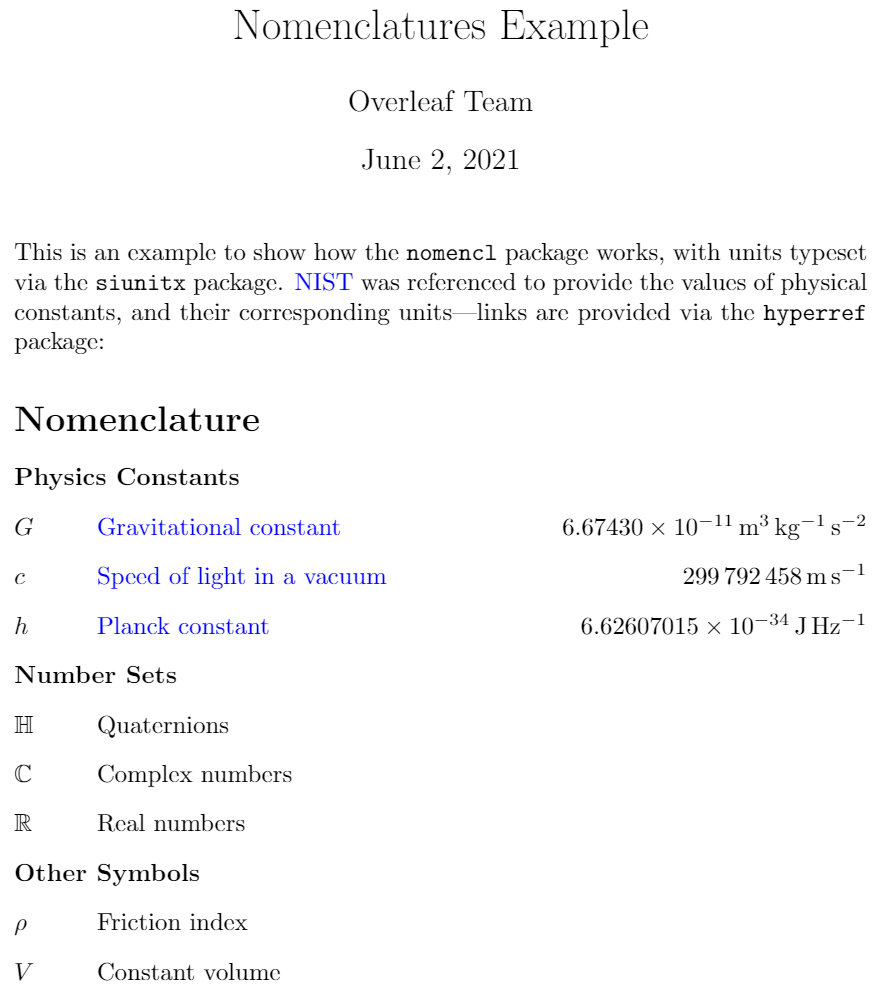## Sorting the Entries

This is the default sorting order:

\documentclass{article}
\usepackage{nomencl}

\makenomenclature

\begin{document}
Here is an example:

\nomenclature{$$+a$$}{Operator}
\nomenclature{$$2a$$}{Number}
\nomenclature{$$:a$$}{Punctuation symbol}
\nomenclature{$$Aa$$}{Uppercase letter}
\nomenclature{$$aa$$}{Lowercase letter}
\nomenclature{$$\alpha$$}{Greek character}

\printnomenclature

\end{document}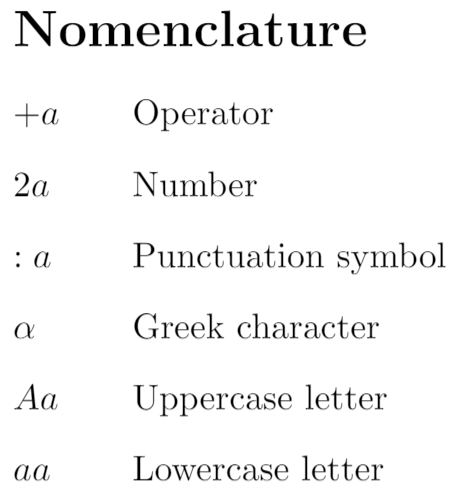Notice that the Greek character showed up before the alphabetic characters because of the backslash \ in \alpha.

Just like for grouping, it is possible to use a prefix to manually sort the nomenclature entries:

\documentclass{article}
\usepackage{nomencl}

\makenomenclature

\begin{document}
Here is an example:

\nomenclature{$$+a$$}{Operator}
\nomenclature{$$2a$$}{Number}
\nomenclature{$$:a$$}{Punctuation symbol}
\nomenclature{$$Aa$$}{Uppercase letter}
\nomenclature{$$aa$$}{Lowercase letter}
\nomenclature{$$\alpha$$}{Greek character}

\printnomenclature

\end{document}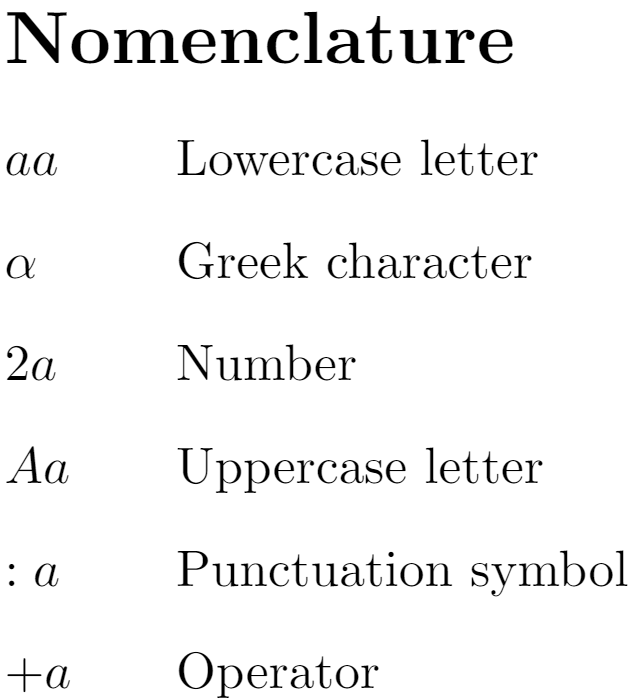The number inside the brackets determines the order to print the corresponding symbol. One can also combine grouping and manually sorting:

\documentclass{article}
\usepackage{amssymb}
\usepackage{nomencl}
\makenomenclature

\usepackage{etoolbox}
\renewcommand\nomgroup{%
\item[\bfseries
\ifstrequal{#1}{A}{Physics Constants}{%
\ifstrequal{#1}{B}{Number Sets}{%
\ifstrequal{#1}{C}{Other Symbols}{}}}%
]}

\begin{document}
Here is an example:

\nomenclature[A, 02]{$$c$$}{Speed of light in a vacuum}
\nomenclature[A, 03]{$$h$$}{Planck constant}
\nomenclature[A, 01]{$$G$$}{Gravitational constant}
\nomenclature[B, 03]{$$\mathbb{R}$$}{Real numbers}
\nomenclature[B, 02]{$$\mathbb{C}$$}{Complex numbers}
\nomenclature[B, 01]{$$\mathbb{H}$$}{Octonions}
\nomenclature[C]{$$V$$}{Constant volume}
\nomenclature[C]{$$\rho$$}{Friction index}

\printnomenclature

\end{document}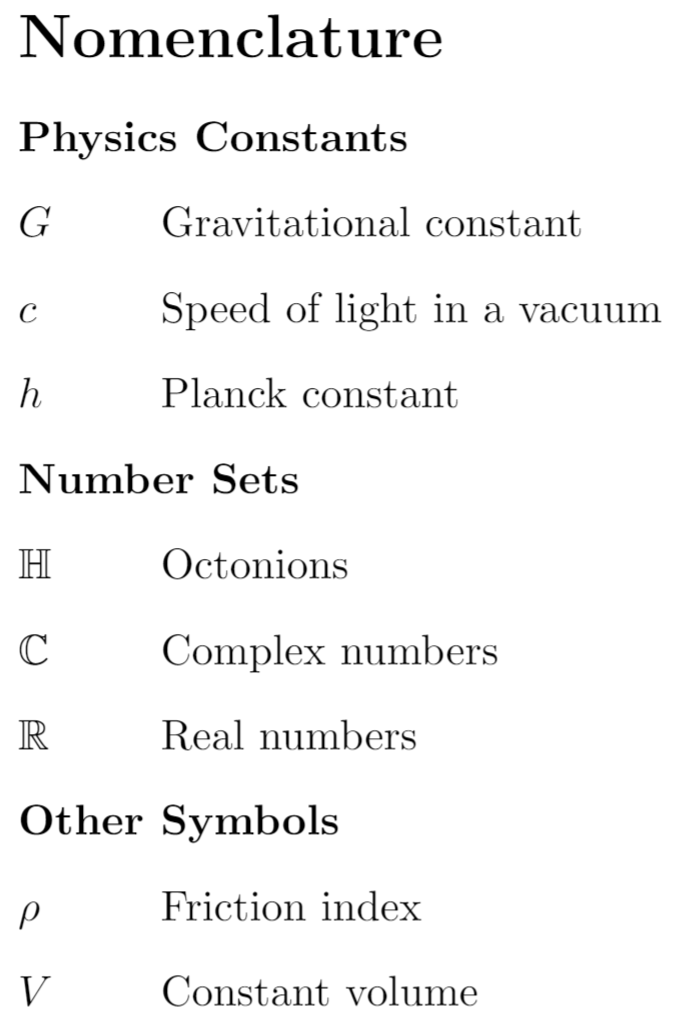Notice that the capital letters used for grouping are different from the ones used in the example at previous section, because that letter is used to sort the groups.